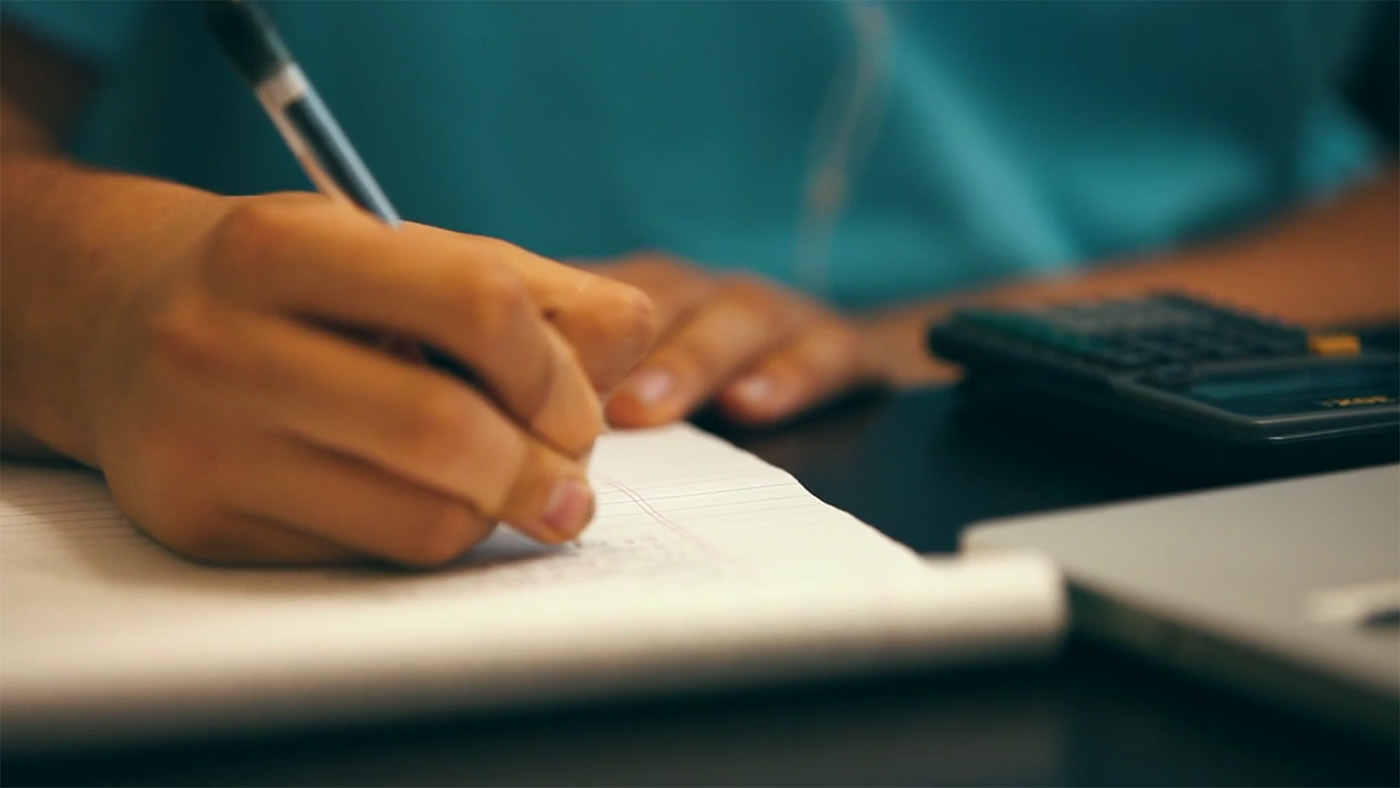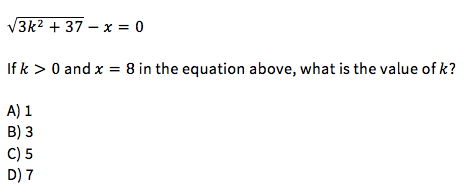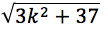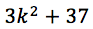# Tests Uncovered: No-Calculator Section on Redesigned SAT MathThis week we are taking a look at how to approach the Redesigned SAT No Calculator Math Section coming March 2016.

#### What does this section look like?

The No Calculator Section has 20 questions to be completed in 25 minutes.

Multiple-Choice Questions                                15
Student-Produced Responses (Grid-Ins)       5

TOTAL QUESTIONS                                             20

Like the Calculator Section, the No Calculator Section begins with a reference box including several relevant math formulas and figures.

#### How much of the test is No Calculator?

The No Calculator Section contains fewer questions than the Calculator Section. As a result, the No Calculator Section only affects 34% of your total Math score on the new SAT.

#### How does this compare to the current SAT?

Students are permitted to use approved calculators for all Math questions on the current SAT. On the redesigned SAT, there will be two different types of Math sections: one permitting the use of a calculator and one prohibiting it.

#### What does this mean for students?

While the concept of “No Calculator” may sound intimidating, there are a few concrete steps students can take to improve their performance in this section:

Write it out

This advice is especially true for word problems. Here, the writers will present you with a lot of information at once. Read the problem sentence by sentence and write down what you know as you go.

Master the Basics
Practice outside the SAT. You may be surprised by how much you’ve been relying on your calculator in everyday classes. Try practicing math in or out of school without a calculator. The more you practice, the faster and more accurate you will become.

Work Backwards
Problems on the Redesigned SAT will try to trick you into doing a lot of complicated math. However, this is rarely (if ever) the most efficient way to solve a No Calculator SAT problem. Instead, try testing the answer choices directly.

Example:Now, this problem could be solved with algebra. However, it would be faster (and easier) to test each answer choice directly. Start by replacing x with 8 in the problem. Move it to the other side of the equation and noticemust equal 8. That meansmust equal 64. Simply replace k with each answer choice until you find 64. The correct answer to this problem is (B).

#### Will the No Calculator Section be on the PSAT?

Yes. Every PSAT will also include a No Calculator Section. It will be 1 of 2 Math sections and form 35% of your PSAT Math score.